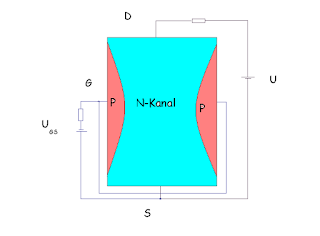# Field effect transistor (FET) | JFET | UJT | BJT

## Field effect transistor (FET)

The field effect transistor is also known as junction field effect transistor it is a unipolar voltage controller device in which output current is control by the input voltage FET is also useful in the amplification purpose similar to the p-n-p or n-p-n transistor however these are basic difference between normal transistor or bipolar junction transistor (BJT) and field effect transistor which are as followField effect transistor (FET) | JFET | UJT | BJT

### UJT (unipolar junction transistor, FET)

1-The word UJT stands for unipolar junction transistor i.e. a transistor in which current flow due to one type of charge carrier only
2-In UJT the output current is control by the input current
3-In UJT there are three terminal Gate, Source and Drain
4-In UJT the input terminal remains in reverse bias condition hence the input impedance of UJT is very high
5-In UJT the Drain and source terminal may be exchange

### BJT [Bipolar junction transistor/ normal transistor (n-p-n/p-n-p transistor)]

The word BJT stands for bipolar junction transistor i.e. a transistor in which current flow through both type of charge carrier electron and holes
1-In BJT the output current is control by the input current applied across input terminal of BJT
2-In BJT there are also three terminal Emitter, Base and collector respectively
3-In BJT the input terminal remain in forward bias condition hence the input impedance remain very small
4-In BJT emitter and collector terminal are not exchange
5-Junction field effect transistor (JFET)

The field effect transistor is also known as junction field effect transistor which is voltage control device i.e. the output current (drain current) is control by the input voltage i.e. the reverse voltage applied across gate and source terminal the symbolic representation of JFET is shown below

Depending on the popularity of charge carrier there are two type of JFET these are n-channel JFET and p-channel JFET both JFET are differ only by their charge carrier participate in current conduction
Constriction of JFET
To construct a junction field effect transistor a rectangular subtract of doped semiconductor i.e. p-type doped in p-channel JFET and n-type doped in n-channel JFET as used, From opposite two side of doped subtract the two terminal are taken known as drain and source across the drain and source the two opposite type doped region are constracted